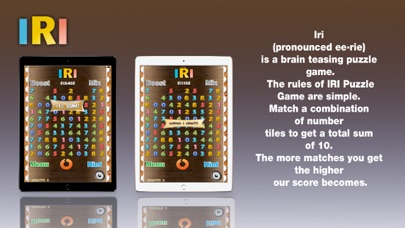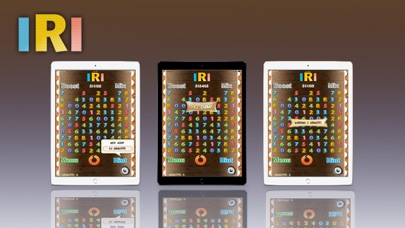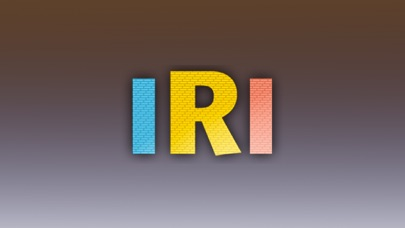# IRI (ee-rie)

## iOS UniversalGames

Free was 2.99

Iri (pronounced ee·rie) is a brain teasing puzzle game. The rules of IRI Puzzle Game are simple. Match a combination of number tiles to get a total sum of 10. The more matches you get the higher your score becomes

Originally a Pontaba, Inc game, the rules of IRI Puzzle Game are simple. Match a combination of number tiles to get a total sum of 10. The number you make equals your score. For example: 2+1+3+1+3=10 (21,313 points), 8+2 = 10 (82 points), 2+2+2+2+1+0+0+1=10 (22,221,001 points). When you get stuck, use game earned credits to rotate the play field to mix-up the number tiles for better matches. It features two modes of play and three ways to help Improve scoring.

•••